Next: 1.3.3 EM Lifetime Extrapolation Up: 1.3 Empirical and Semi-Empirical Previous: 1.3.1 Black's Equation

## 1.3.2 Resistance Increase and Void Growth

EM experiments normally use a given resistance increase as failure criterion. For a void spanning the entire cross sectional area of a line, the total resistance of a damascene line, as shown in Figure 1.5, is given by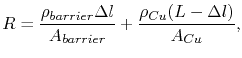(1.8)

where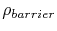is the electrical resistivity and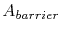is the cross sectional area of the barrier layer,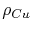is the electrical resistivity and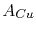is the cross sectional area of the copper line,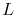is the line length, and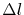is the length of the void. Thus, this equation relates resistance increase with void size. In turn, void size is connected to mass transport, which is expressed in the form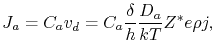(1.9)

whereis the atomic concentration,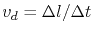is the drift velocity,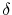is the width of the interface which controls mass transport,is the line height,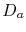is the atomic diffusivity of the interface which dominates the EM transport,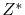is the effective valence,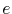is the elementary charge,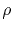is the electrical resistivity, and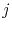is the current density.The atomic flux can also be written as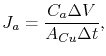(1.10)

where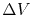is the change of the void volume in a time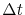. Combining (1.9) and (1.10) yields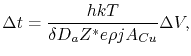(1.11)

so that a given test time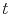is related to the void volume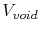,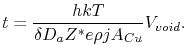(1.12)

Equation (1.12) is commonly used for estimation of the void growth time. In turn, a critical void volume is related to the line resistance according to (1.8). Thus, the time to failure for a given resistance increase criterion can be determined.

An interesting application of such an approach was performed by Hauschildt et al. [20,22]. For a void spanning the entire line width,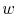, and assuming a rectangular void shape, the void volume becomes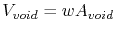, and (1.12) yields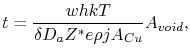(1.13)

where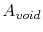is the area of the void, which can be measured using SEM pictures. EM tests were then carried out and stopped after a given test time (called t-based by the authors) or after a certain resistance increase (R-based). In both cases, the void area mean value and standard deviation were determined. For the R-based tests the mean time to failure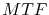can be determined which, in turn, can be used for the t-based experiments. Finally, using the void area statistical distribution of both tests and applying (1.13) a new distribution is obtained following [20,22]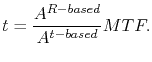(1.14)

Hence, once the statistics of the void area distribution for both test types is known, the distribution of the EM lifetimes can be estimated.

Equation (1.9) is also commonly used to extract the diffusivity from void drift experiments . The drift velocity of the void boundary in Figure 1.5 is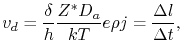(1.15)

which for a given test time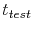with a corresponding void length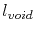can be rearranged as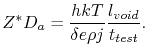(1.16)

Sincecan be expressed by an Arrhenius relationship, the activation energy for diffusion can be extracted from a plot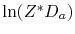versus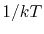.Next: 1.3.3 EM Lifetime Extrapolation Up: 1.3 Empirical and Semi-Empirical Previous: 1.3.1 Black's Equation

R. L. de Orio: Electromigration Modeling and Simulation